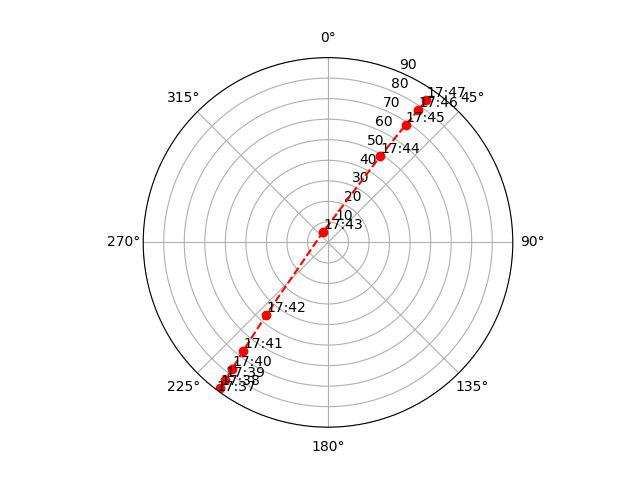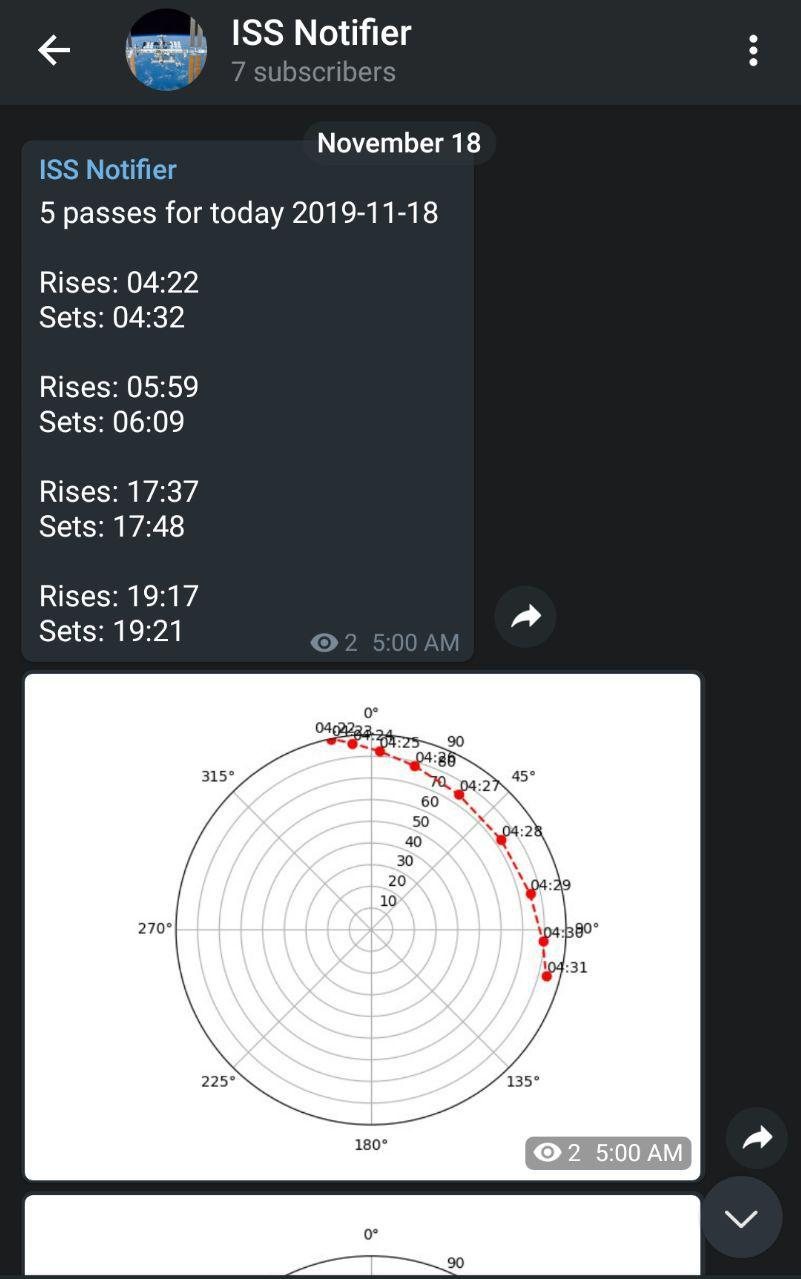The ISS orbits the earth aproximately 16 times per day. This means that if you look at the sky at the right time you can see it as a bright star moving fast across the horizion

I looked at apps that would remind me when iss passes visible over me but found none that did the job well enough. So i set out to build my own tool that will show when i should look up

### ISS botEach day,the bot checks whether there are any visible passes if so texts to the telegram channel.

The source code for it is available here

## How it works

Part of the main function which does calculations

``````    stations_url = 'http://celestrak.com/NORAD/elements/stations.txt'
satellite = satellites['ISS (ZARYA)']

#get values for time,we only search for times in which passes would be visible morning and dusk
#this is due to the sunsbrightness and lackof light to be reflected
minutes1 = range(240,1440) # 4 to 7
minutes2 = range(1050,1170) # 1530 to 1930

minutes = minutes1 #+ list(minutes2)

minutes = range(start,end)
t = ts.utc(dt.year, dt.month, dt.day, -5, minutes)

#pedict according to time
loc = Topos(cords, cords)
difference = (satellite - loc).at(t)
alt, az, distance = difference.altaz()

#Get times itsabove horizon
above_horizon = alt.degrees > 0

``````

### On a high level the script uses python spacefield to

• fetch tles from celestrak

``````stations_url = 'http://celestrak.com/NORAD/elements/stations.txt'
satellite = satellites['ISS (ZARYA)']
``````
• calculate the altiutude , azimuth and distance between the input location and the ISS for the given time range t.(The values of minutes1 and 2 are the ranges of time at which light’ll reflect of the ISS)

``````#get values for time,we only search for times in which passes would be visible morning and dusk
#this is due to the sunsbrightness and lackof light to be reflected
minutes1 = range(240,1440) # 4 to 7
minutes2 = range(1050,1170) # 1530 to 1930

minutes = minutes1 #+ list(minutes2)

minutes = range(start,end)
t = ts.utc(dt.year, dt.month, dt.day, -5, minutes)

#pedict according to time
loc = Topos(cords, cords)
difference = (satellite - loc).at(t)
alt, az, distance = difference.altaz()
``````
• Finds the values of t at which ISS has a positive altitude

``````#Get times itsabove horizon
above_horizon = alt.degrees > 0

above_horizon[-1] = 0
above_horizon = 0
#above_horizon = insert(above_horizon,-1,0)

#boundaries by setsand rising time
boundaries, = diff(above_horizon).nonzero()

``````